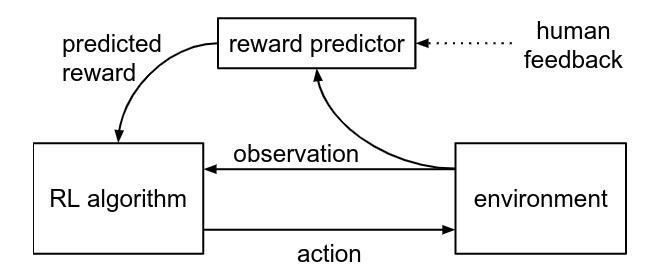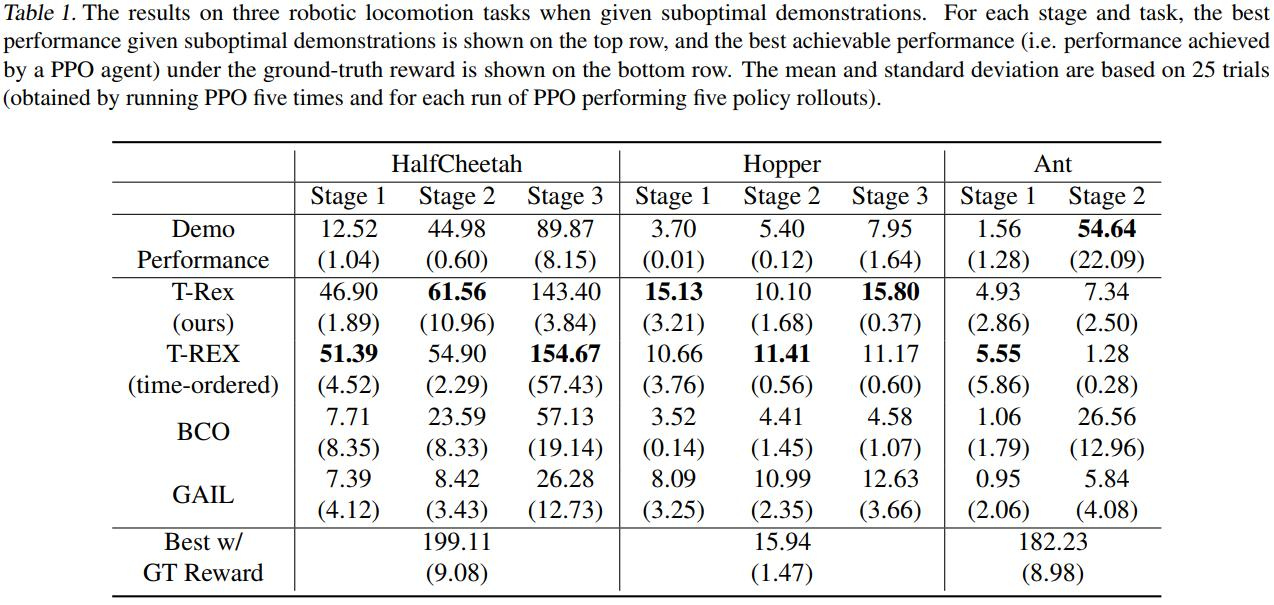## Introduction

Inverse-Reinforcement Learning methods are usually bounded by the expertise of the demonstrator as they presume the demonstrator is optimal. The authors propose a way to circumvent this limitation by training a neural network how to tell which demonstrations are better than others. This allows the learned reward to extrapolate beyond the demonstrations and become better than the demonstrators.

## Methods

The article follows closely  where demonstrations are “ranked” by human “jugdes”.where the reward predictor is a neural network. A trajectory $$\sigma^1$$ is prefered over trajectory $$\sigma^2$$ if

$(o^{1}_{0}, o^{1}_{1}, ..., o^{1}_{t}) \succ (o^{2}_{0}, o^{2}_{1}, ..., o^{2}_{t}) \rightarrow r(o^{1}_{0}) + ... + r(o^{1}_{t}) > r(o^{2}_{0}) + ... + r(o^{2}_{t})$

where $$o$$ is an observation. The reward network $$\hat{r}$$ is then trained with the following cross-entropy-style loss:

$\mathcal{L(\hat{r}} = \sum_{(\sigma^1, \sigma^2, \mu) \in \mathcal{D}} \mu log \hat{P} [\sigma^1 \succ \sigma^2] + (1-\mu) log \hat{P} [sigma^2 \succ sigma^1]$

where

$\hat{P} [\sigma^1 \succ \sigma^2] = \dfrac{exp\sum{}\hat{r}(o^1_t)}{exp\sum{}\hat{r}(o^1_t) + exp\sum{}\hat{r}(o^2_t)}$

The main difference between  and this article is that instead of asking humans to rate the generated trajectories, the authors assumed that a model trained to mimic a not-necessarily-optimal expert would get better over time and therefore produce increasingly better trajectories itself.

## Experiments

The RL algorithm used was PPO, and to generate demonstrations, they trained agents using PPO and the ground truth reward and kept the trajectories at three different “stages”1: Deep reinforcement learning from human preferences, Christiano, P. F., Leike, J., Brown, T., Martic, M., Legg, S., and Amodei, D